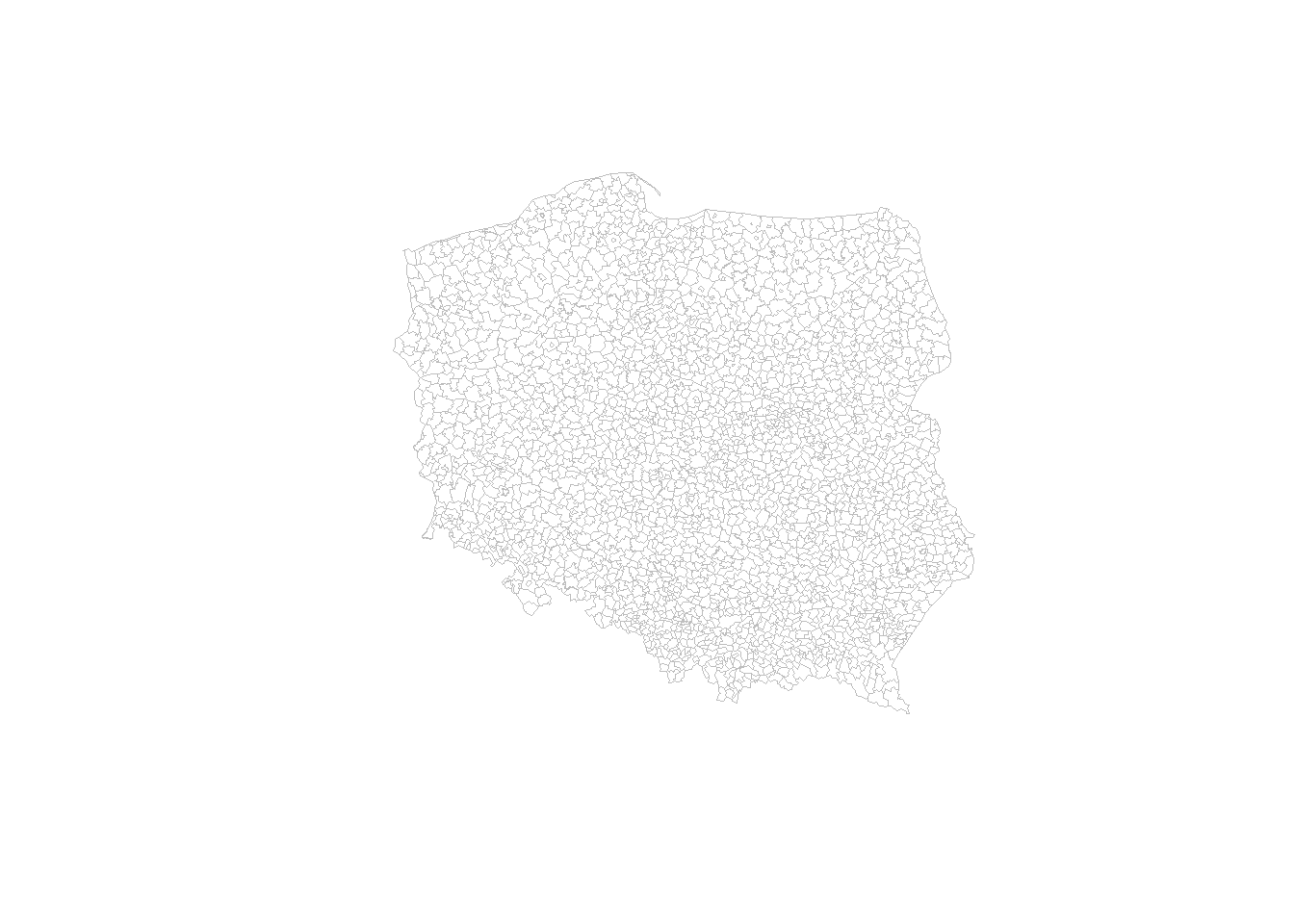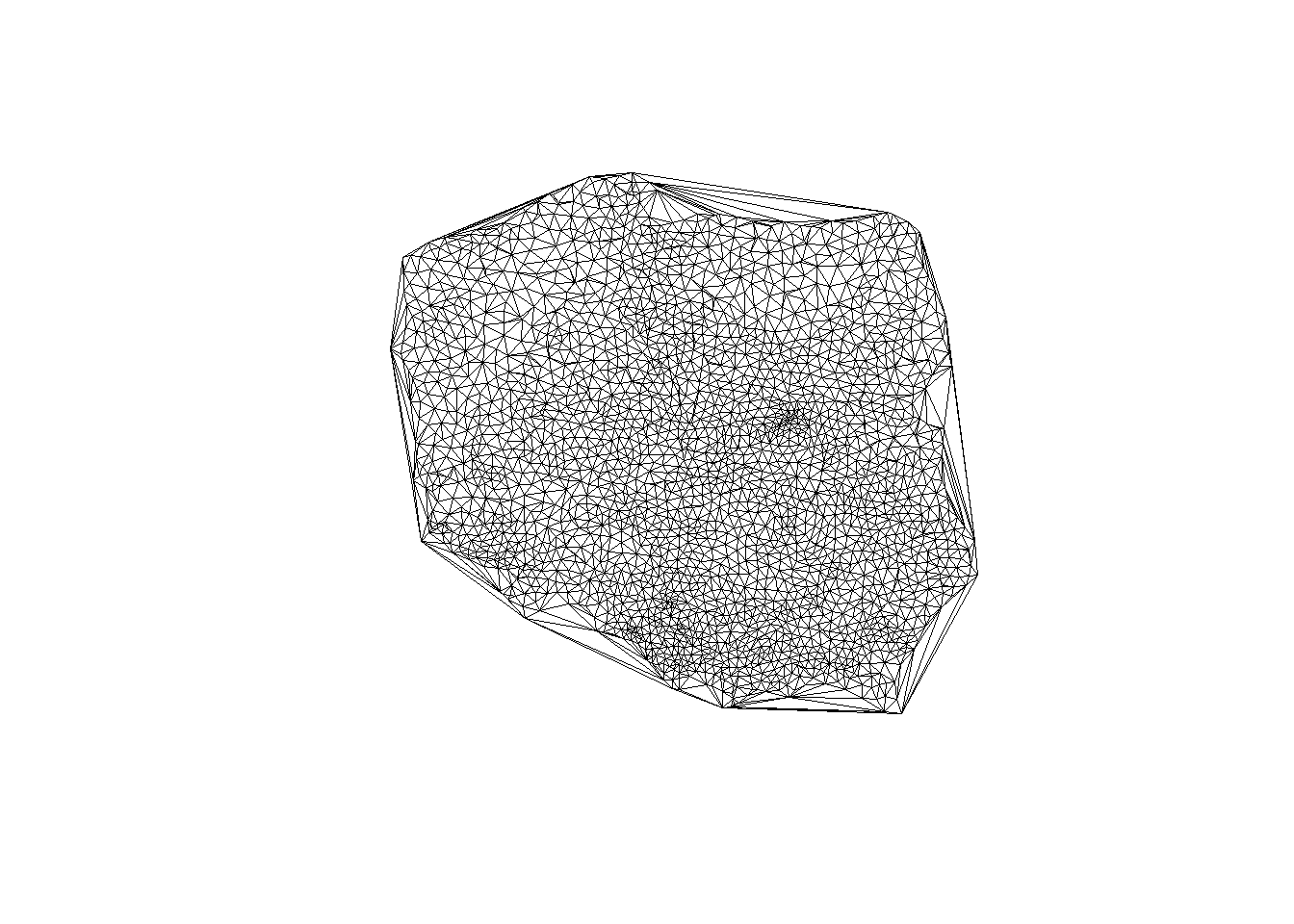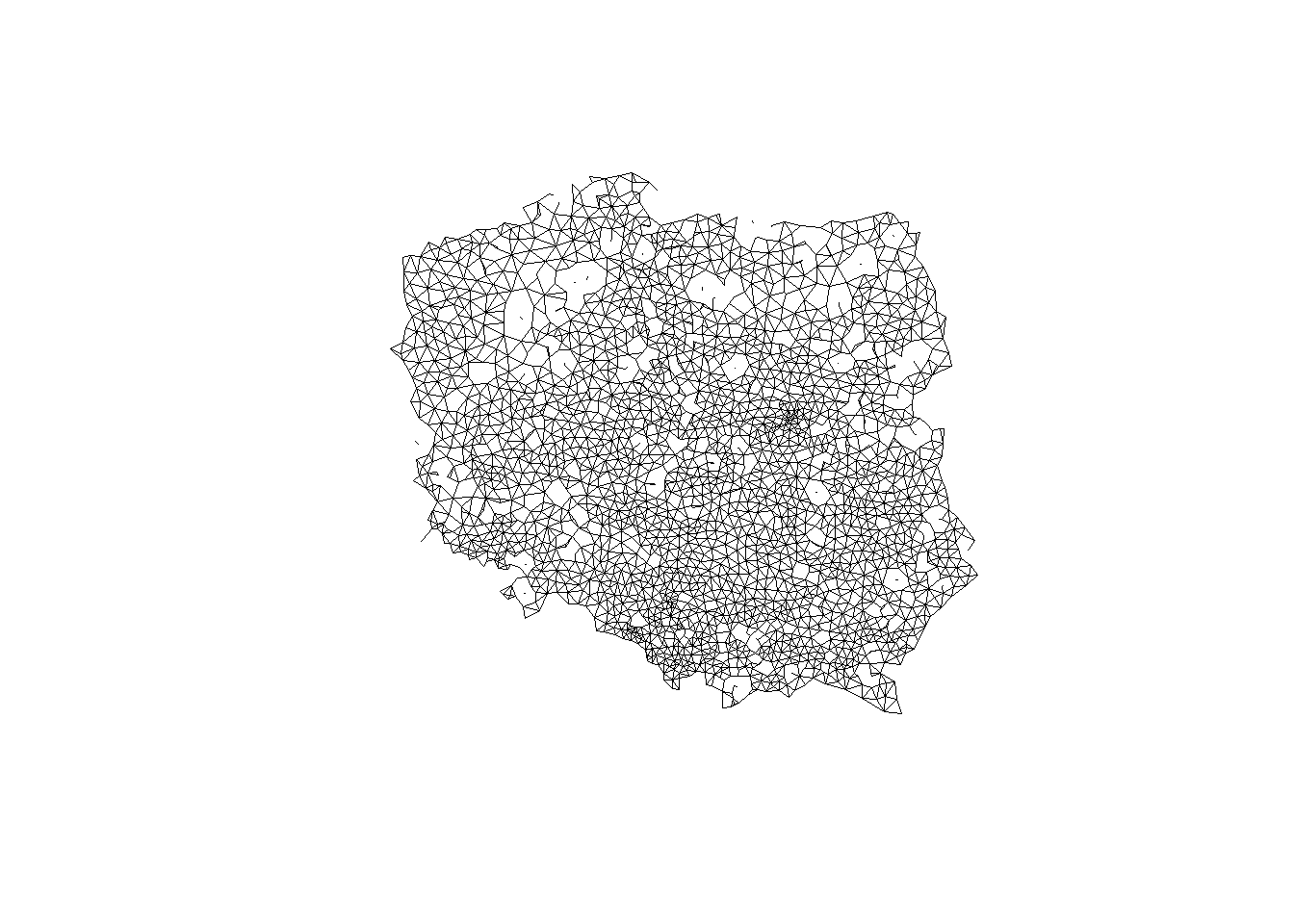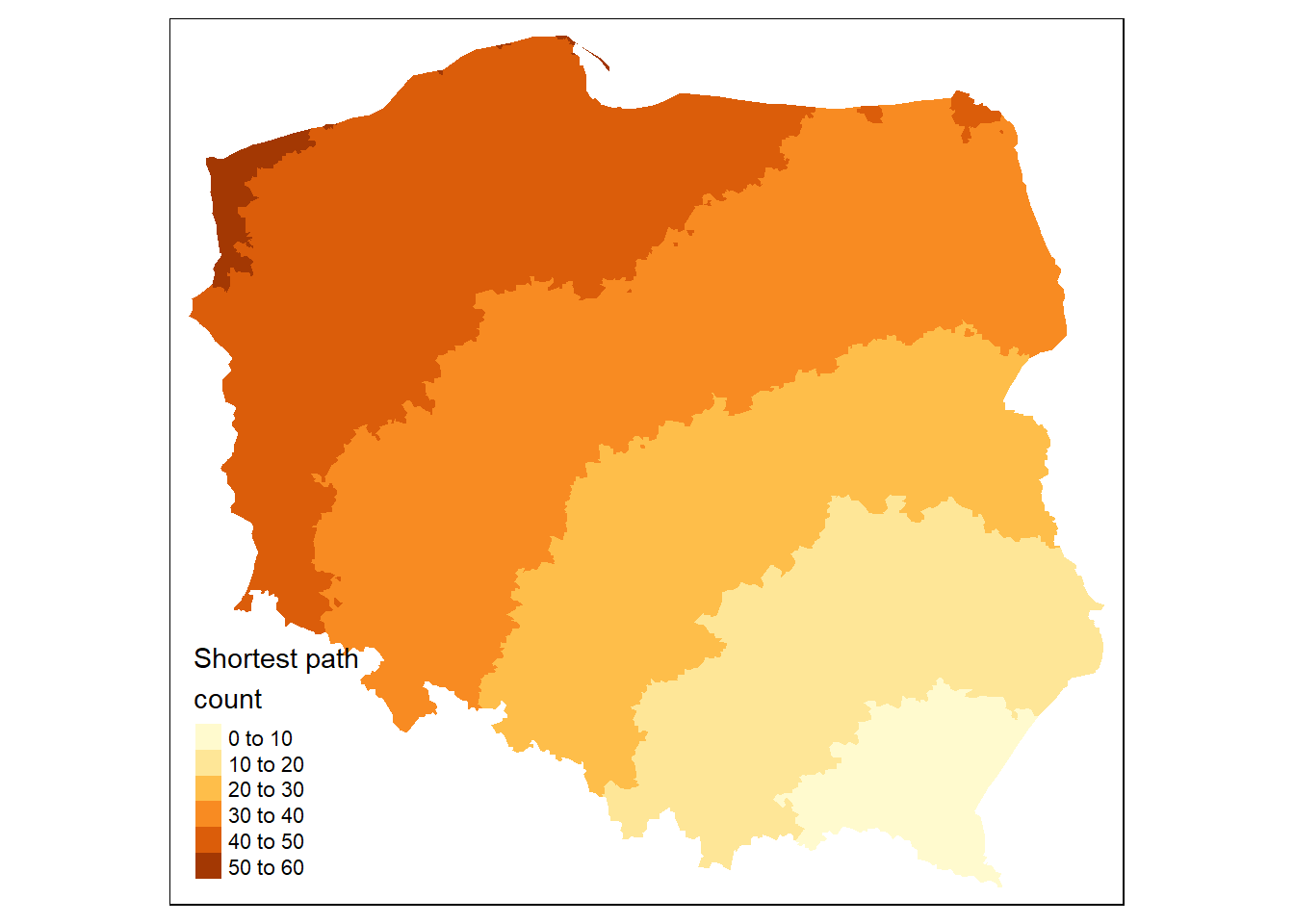# Proximity and Areal Data

Learning objectives:

• consider the definition of areal data
• discuss matters of graph theory
• explore various methods of finding neighbors
library("dbscan")     #density-based clustering
library("igraph")     #graph networks
library("Matrix")     #for sparse representation
library("sf")         #simple features
library("spatialreg") #spatial regression analysis
library("spdep")      #spatial dependence
library("tmap")       #quick, thematic maps

data(pol_pres15, package = "spDataLarge") #Poland 2015 election data

sessionInfo()
R version 4.3.0 (2023-04-21 ucrt)
Platform: x86_64-w64-mingw32/x64 (64-bit)
Running under: Windows 10 x64 (build 19045)

Matrix products: default

locale:
 LC_COLLATE=English_United States.utf8
 LC_CTYPE=English_United States.utf8
 LC_MONETARY=English_United States.utf8
 LC_NUMERIC=C
 LC_TIME=English_United States.utf8

time zone: America/Los_Angeles
tzcode source: internal

attached base packages:
 stats     graphics  grDevices utils     datasets  methods   base

other attached packages:
 tmap_3.3-3       spdep_1.2-8      spatialreg_1.2-9 spData_2.2.2
 sf_1.0-14        Matrix_1.5-4     igraph_1.4.3     dbscan_1.1-11

loaded via a namespace (and not attached):
 raster_3.6-23      xfun_0.40          htmlwidgets_1.6.2  lattice_0.21-8
 vctrs_0.6.3        tools_4.3.0        crosstalk_1.2.0    LearnBayes_2.15.1
 generics_0.1.3     parallel_4.3.0     sandwich_3.0-2     tibble_3.2.1
 proxy_0.4-27       fansi_1.0.4        pkgconfig_2.0.3    KernSmooth_2.23-20
 RColorBrewer_1.1-3 leaflet_2.1.2      lifecycle_1.0.3    compiler_4.3.0
 deldir_1.0-9       terra_1.7-46       codetools_0.2-19   leafsync_0.1.0
 stars_0.6-3        htmltools_0.5.6    class_7.3-21       pillar_1.9.0
 MASS_7.3-58.4      classInt_0.4-10    lwgeom_0.2-13      wk_0.8.0
 boot_1.3-28.1      abind_1.4-5        multcomp_1.4-25    nlme_3.1-162
 tidyselect_1.2.0   digest_0.6.33      mvtnorm_1.2-0      dplyr_1.1.3
 splines_4.3.0      fastmap_1.1.1      grid_4.3.0         expm_0.999-7
 cli_3.6.1          magrittr_2.0.3     base64enc_0.1-3    dichromat_2.0-0.1
 XML_3.99-0.14      survival_3.5-5     utf8_1.2.3         leafem_0.2.0
 TH.data_1.1-2      e1071_1.7-13       sp_2.0-0           spDataLarge_2.0.9
 rmarkdown_2.24     png_0.1-8          zoo_1.8-12         coda_0.19-4
 evaluate_0.21      knitr_1.43         tmaptools_3.1-1    viridisLite_0.4.2
 s2_1.1.4           rlang_1.1.1        Rcpp_1.0.11        glue_1.6.2
 DBI_1.1.3          rstudioapi_0.15.0  jsonlite_1.8.7     R6_2.5.1
 units_0.8-3       

## Areal Data

Areal units of observation are very often used when simultaneous observations are aggregated within non-overlapping boundaries.

Example: Lung cancer SIR (standardized incidence rate) in Pennsylvania

## Proximity Data

By proximity, we mean closeness in ways that make sense for the data generation processes thought to be involved. In cross-sectional geostatistical analysis with point support, measured distance makes sense for typical data generation processes.

Example: Voronoi diagram of Pennsylvania

## Support

By support of data we mean the physical size (length, area, volume) associated with an individual observational unit

• It is possible to represent the support of areal data by a point, despite the fact that the data have polygonal support
• When the intrinsic support of the data is represented as points, but the underlying process is between proximate observations rather than driven chiefly by distance between observations
• risk of misrepresenting the footprint of the underlying spatial processes

## Representing Proximity

Ideas for spatial autocorrelation

• (graph theory) undirected graph, and its neighbors, or
• (geospatial) variogram

• islands?
• disconnected subgraphs?
• sparse areas (cutoff by distance threshold)

## spdep package

spdep package: spatial dependence

• nb class for neighbor

• list of length 0L for no neighbors
• listw object

1. nb object
2. list of numerical weights
3. how the weights were calculated
• spatialreg package now has the functions for constructing and handling neighbour and spatial weights objects, tests for spatial autocorrelation, and model fitting functions that used to be in spdep

## Example: Poland 2015 Election

pol_pres15 |>
subset(select = c(TERYT, name, types)) |>
head()
Simple feature collection with 6 features and 3 fields
Geometry type: MULTIPOLYGON
Dimension:     XY
Bounding box:  xmin: 235157.1 ymin: 366913.3 xmax: 281431.7 ymax: 413016.6
Projected CRS: ETRS89 / Poland CS92
TERYT                name       types                       geometry
1 020101         BOLESŁAWIEC       Urban MULTIPOLYGON (((261089.5 38...
2 020102         BOLESŁAWIEC       Rural MULTIPOLYGON (((254150 3837...
3 020103            GROMADKA       Rural MULTIPOLYGON (((275346 3846...
4 020104        NOWOGRODZIEC Urban/rural MULTIPOLYGON (((251769.8 37...
5 020105          OSIECZNICA       Rural MULTIPOLYGON (((263423.9 40...
6 020106 WARTA BOLESŁAWIECKA       Rural MULTIPOLYGON (((267030.7 38...
# tmap
tm_shape(pol_pres15) + tm_fill("types")For safety’s sake, we impose topological validity:

if (!all(st_is_valid(pol_pres15)))
pol_pres15 <- st_make_valid(pol_pres15)

## Contiguous Neighbors

For each observation, the poly2nb function checks whether

• at least one (queen = TRUE)
• at least two (“rook”, queen = FALSE)

points are within snap distance of each other.

pol_pres15 |> poly2nb(queen = TRUE) -> nb_q
print(nb_q)
Neighbour list object:
Number of regions: 2495
Percentage nonzero weights: 0.2287862
Average number of links: 5.708216 
• s2 spherical coordinates are used by default
• symmetric relationships assumed
• row.names may be customized

### Connected

Are the data connected? (Some model estimation techniques do not support graphs that are not connected.)

(nb_q |> n.comp.nb())$nc # result: 1 for TRUE (more than one for FALSE) verbose code #library(Matrix, warn.conflicts = FALSE) #library(spatialreg, warn.conflicts = FALSE) nb_q |> nb2listw(style = "B") |> as("CsparseMatrix") -> smat #library(igraph, warn.conflicts = FALSE) smat |> graph.adjacency() -> g1 g1 |> count_components()  1 ## Graph-Based Neighbors The simplest form is by using triangulation, here using the deldir package # get centroids and save their coordinates pol_pres15 |> st_geometry() |> st_centroid(of_largest_polygon = TRUE) -> coords # triangulation (coords |> tri2nb() -> nb_tri) Neighbour list object: Number of regions: 2495 Number of nonzero links: 14930 Percentage nonzero weights: 0.2398384 Average number of links: 5.983968  ### How Far Away are the Neighbors? # results in meters nb_tri |> nbdists(coords) |> unlist() |> summary()  Min. 1st Qu. Median Mean 3rd Qu. Max. 246.6 9847.2 12151.2 13485.2 14993.5 296973.7  ### Sphere of Influence The Sphere of Influence soi.graph function takes triangulated neighbours and prunes off neighbour relationships represented by edges that are unusually long for each point. (nb_tri |> soi.graph(coords) |> graph2nb() -> nb_soi) Neighbour list object: Number of regions: 2495 Number of nonzero links: 12792 Percentage nonzero weights: 0.2054932 Average number of links: 5.127054  # Poland pol_pres15 |> st_geometry() |> plot(border = "grey", lwd = 0.5)# triangulation plot(nb_tri, coords = coords, points = FALSE, lwd = 0.5)# sphere of influence plot(nb_soi, coords = coords, points = FALSE, lwd = 0.5)## Distance-Based Neighbors • Distance-based neighbours can be constructed using dnearneigh, with a distance band with lower d1= and upper d2= bounds controlled by the bounds= argument • The knearneigh function for -nearest neighbours returns a knn object, converted to an nb object using knn2nb • Computation speed boost through dbscan package • The nbdists function returns the length of neighbour relationship edges coords |> knearneigh(k = 1) |> knn2nb() |> nbdists(coords) |> unlist() |> summary()  Min. 1st Qu. Median Mean 3rd Qu. Max. 246.5 6663.4 8538.0 8275.1 10123.9 17978.8  Here the largest first nearest neighbour distance is just under 18 km, so using this as the upper threshold gives certainty that all units will have at least one neighbour. # maybe no neighbors coords |> dnearneigh(0, 16000) -> nb_d16 # at least one neighbor coords |> dnearneigh(0, 18000) -> nb_d18 • Adding 300 m to the threshold gives us a neighbour object with no no-neighbour units, and all units can be reached from all others across the graph. # connected graph coords |> dnearneigh(0, 18300) -> nb_d183 It is possible to control the numbers of neighbours directly using -nearest neighbours, either accepting asymmetric neighbours coords |> knearneigh(k = 6) -> knn_k6 # asymmetrical knn_k6 |> knn2nb() -> nb_k6 # symmetrical knn_k6 |> knn2nb(sym = TRUE) -> nb_k6s ## Weights Specification Once neighbour objects are available, further choices need to be made in specifying the weights objects. • The nb2listw function is used to create a listw weights object with an nb object, a matching list of weights vectors, and a style specification • Because handling no-neighbour observations now begins to matter, the zero.policy= argument is introduced (default: FALSE) • n: number of observations • $$S_{0}$$: sum of weights The “B” binary style gives a weight of unity to each neighbour relationship, and typically up-weights units with no boundaries on the edge of the study area, having a higher count of neighbours. nb_q |> nb2listw(style = "B") -> lw_q_B lw_q_B |> spweights.constants() |> data.frame() |> subset(select = c(n, S0))  n S0 1 2495 14242 The “W” row-standardised style up-weights units around the edge of the study area that necessarily have fewer neighbours. This style first gives a weight of unity to each neighbour relationship, then it divides these weights by the per unit sums of weights (caution: avoid no-neighbors) nb_q |> nb2listw(style = "W") -> lw_q_W lw_q_W |> spweights.constants() |> data.frame() |> subset(select = c(n, S0))  n S0 1 2495 2495 ### Inverse Distance Weights nb_d183 |> nbdists(coords) |> lapply(function(x) 1/(x/1000)) -> gwts No-neighbour handling is by default to prevent the construction of a weights object, making the analyst take a position on how to proceed. (nb_d183 |> nb2listw(glist=gwts, style="B") -> lw_d183_idw_B) |> spweights.constants() |> data.frame() |> subset(select=c(n, S0))  n S0 1 2495 1841.345 Use can be made of the zero.policy= argument to many functions used with nb and listw objects. (nb_d16 |> nb2listw(style="B", zero.policy=TRUE) |> spweights.constants(zero.policy=TRUE) |> data.frame() |> subset(select=c(n, S0)))  n S0 1 2488 15850 ## Higher-Order Neighbors If we wish to create an object showing to neighbours, where $$i$$ is a neighbour of $$j$$, and $$j$$ in turn is a neighbour of $$k$$, so taking two steps on the neighbour graph, we can use nblag (automatically removes $$i$$ to $$i$$ self-neighbours) nb_q |> nblag(2) -> nb_q2 Returning to the graph representation of the same neighbour object, we can ask how many steps might be needed to traverse the graph? igraph::diameter(g1) #where g1 is a graph object  52 We step out from each observation across the graph to establish the number of steps needed to reach each other observation by the shortest path (creating an $$n \times n$$ matrix sps), once again finding the same maximum count. g1 |> shortest.paths() -> sps sps |> apply(2, max) -> spmax spmax |> max()  52 The municipality with the maximum count is called Lutowiska, close to the Ukrainian border in the far south east of the country. mr <- which.max(spmax) pol_pres15$name0[mr]
 "Lutowiska"
pol_pres15\$sps1 <- sps[,mr]
tm_shape(pol_pres15) +
tm_fill("sps1", title = "Shortest path\ncount")## Meeting Videos

### Cohort 1

Meeting chat log
LOG